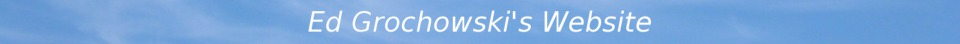## Class AB Biasing

Ed Grochowski

Written 12-21-2022

### Introduction

The class AB audio amplifier uses a pair of output transistors in push-pull configuration. Each transistor conducts during alternate halves of the waveform. Near the zero crossing, both transistors conduct. This article describes how to choose the amount of overlap for minimal distortion.

### Model

The push-pull output stage may be modeled as follows.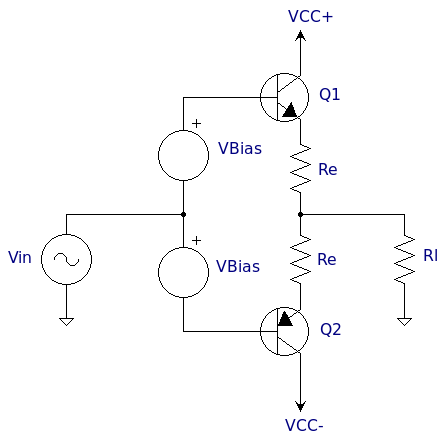The emitter current is an exponential function of base-emitter voltage. This is given by the Ebers-Moll equation (ignoring the insignificant constant term):

```Ie = Is * exp (Vbe/Vt)
```

As the input voltage changes, the current slides up and down the exponential. The push-pull pair has two such exponentials, each a mirror image of the other. The bias determines how far down the exponential the current is allowed to go.

The emitter resistors perform the essential function of making the current less sensitive to Is and temperature. The voltage drop across the resistors is given by Ohm's Law:

```V = I * R
```

Finally, Kirchoff's laws stipulate that the voltage around a loop must sum to zero, and the current into a node must sum to zero.

### Parameters

It is easy to write a simulator that solves the equations numerically. The input is a single-cycle sine wave, and the output is analyzed by FFT.

I chose Is to match the popular 2SC5200. The load resistor is 8 ohms. The model corresponds to one-half of the real amplifier which uses four output transistors to drive a 4 ohm load.

I simulated two common values of emitter resistors, 0.22 ohm and 0.39 ohm. The former leads to lower distortion while the latter leads to more stable bias current. Choosing the value of the emitter resistors requires a separate simulator for thermal stability.

The temperature is 300K.

### Results

The simulation results are shown below.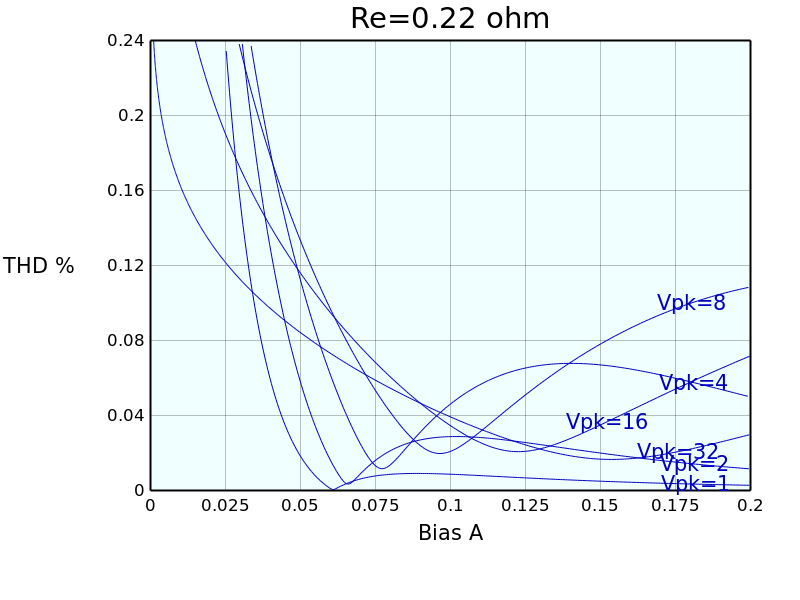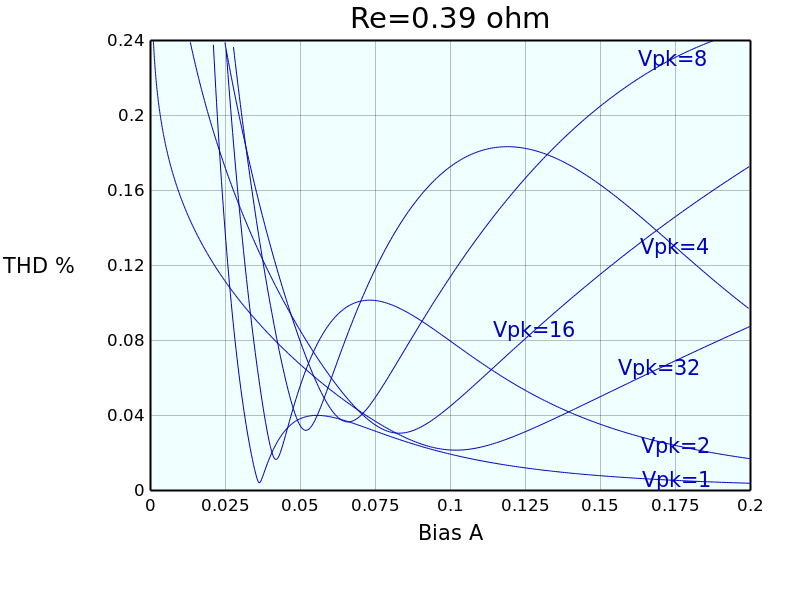The first conclusion is that the emitter-follower output stage is already quite linear. Not much negative feedback is needed to reduce distortion to negligible levels.

The graphs show three regions of operation: underbias, optimal bias, and overbias.

#### Underbias

With very small bias currents (<25mA), the output waveform exhibits a notch around zero volts. Distortion is high. This region must be avoided.

#### Optimal Bias

Between 50mA and 100mA, distortion reaches a local minimum, but it is narrow and hard to realize. In practice, one wants to be on the right side of the minimum.

#### Overbias

With bias currents over 150mA, the behavior differs according to amplitude.

For small amplitudes, increasing the bias current lowers distortion because the transistors are operating over a smaller part of the exponential. This is where class A amplifiers operate.

For large amplitudes, the opposite happens: increasing the bias current increases distortion in class AB because the exponential is causing larger voltage errors in the emitter resistors. This region too must be avoided.

### Implications

The bias currents for minimal distortion require only a few watts of idle power consumption. Moreover, the idle power does not add to the maximum power dissipation because very little time is spent with both transistors conducting at amplitudes that cause maximum dissipation (64% of Vcc).

In addition to the exponential relationship between current and voltage, real transistors exhibit HFE variations with current, and also dynamic effects. With good design, I believe the latter can hide behind the exponential as contributors to distortion.

Setting Re=0 shows that the local minimum and maximum are caused by the non-linear terms in the exponential leading to error voltages in the emitter resistors. These error voltages may result in helpful cancellation of distortion products or harmful contribution.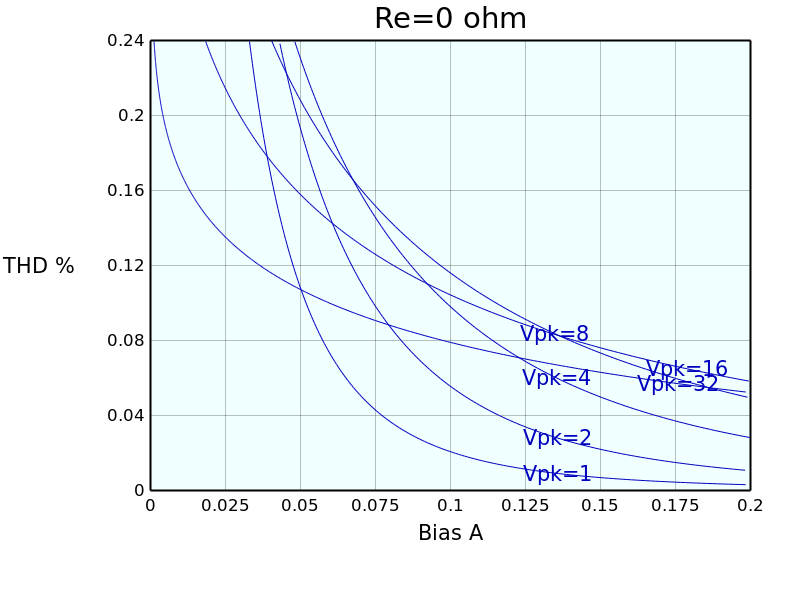Another interesting comparison is with a push-pull pair of MOSFETs. The MOSFET is governed by a square-law relationship between current and voltage:

```Id = Idss * (Vgs - Vth)^2
```

I chose Idss and Vth to match the 2SK1058. Note that the scales have changed.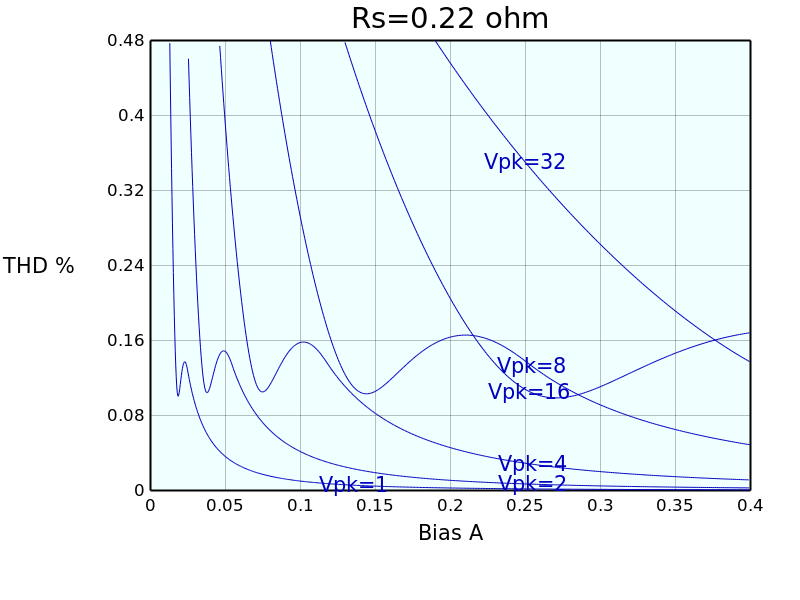The MOSFET's distortion is highest at maximum amplitude. Compared to the bipolar transistor, the MOSFET requires much higher bias current to reach low levels of distortion.